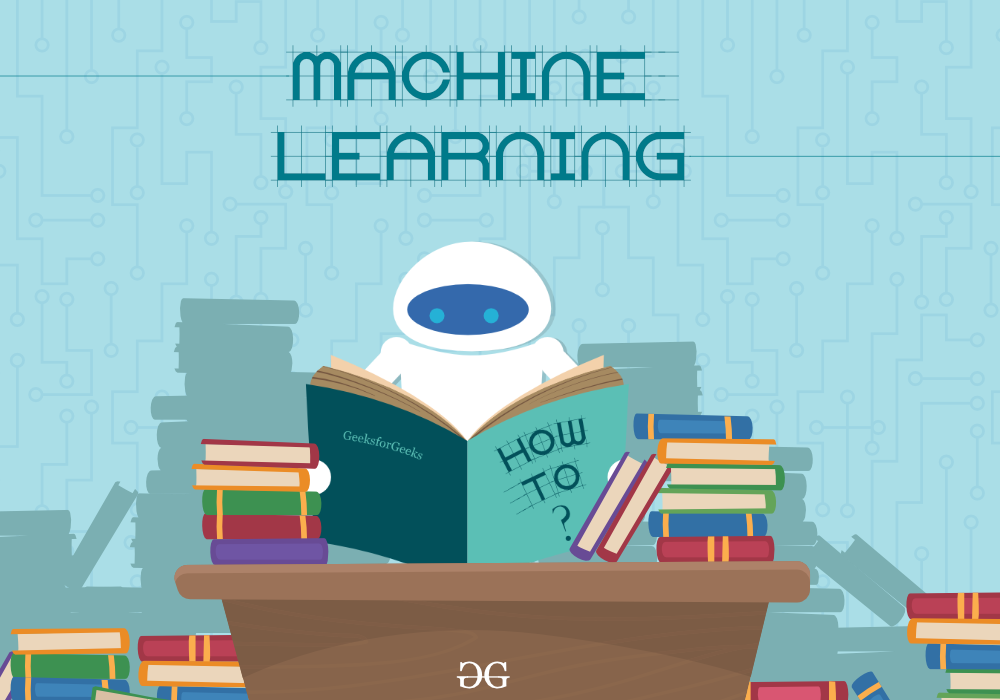GeeksforGeeks App
Open AppBrowser
Continue

# Machine Learning Mathematics

Machine Learning is the field of study that gives computers the capability to learn without being explicitly programmed. Math is the core concept in machine learning which is used to express the idea within the machine learning model.Mathematics for Machine Learning

In this tutorial, we will look at different mathematics concepts and will learn about these modules from basic to advance with the help particular algorithm.

## Linear Algebra and Matrix

Linear Algebra is an algebra extension to an undefined number of dimensions. Linear Algebra concerns the focus on linear equation systems.

## Statistics

Statistics is the collection of data, tabulation, and interpretation of numerical data, and it is applied mathematics concerned with data collection analysis, interpretation, and presentation.

## Geometry

Geometry is the branch of mathematics that deals with the forms, angles, measurements, and proportions of ordinary objects.

## Calculus

Calculus is a subset of mathematics concerned with the study of continuous transition. Calculus is also known as infinitesimal calculus or “infinite calculus.” The analysis of continuous change of functions is known as classical calculus

## Probability and Distributions

Probability and distributions are statistical functions that describe all the possible values.

## Regression

Regression is a statistical process for estimating the relationships between the dependent variables or criterion variables

## Dimensionality Reduction

Dimensionality reduction is a technique to reduce the number of input variables in training data.

My Personal Notes arrow_drop_up